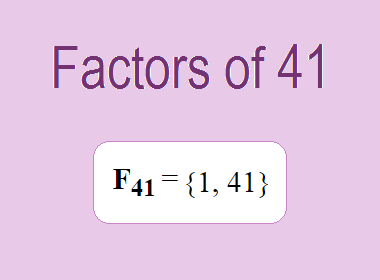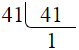# Factors of 41The factors of 41 are 1 and 41 i.e. F41 = {1, 41}. The factors of 41 are all the numbers that can divide 41 without leaving a remainder. 41 is a prime number, so it is divisible by 1 and 41 only.

We can check if these numbers are factors of 41 by dividing 41 by each of them. If the result is a whole number, then the number is a factor of 41. Let's do this for each of the numbers listed above:

·        1 is a factor of 41 because 41 divided by 1 is 41.

·        41 is a factor of 41 because 41 divided by 41 is 1.

## Properties of the Factors of 41

The factors of 41 have some interesting properties. One of the properties is that the sum of the factors of 41 is equal to 42. We can see this by adding all the factors of 41 together:

1 + 41 = 42

Another property of the factors of 41 is that the only prime factor of 41 is 41 itself.

********************

********************

## Applications of the Factors of 41

The factors of 41 have several applications in mathematics. One of the applications is in finding the highest common factor (HCF) of two or more numbers. The HCF is the largest factor that two or more numbers have in common. For example, to find the HCF of 41 and 82, we need to find the factors of both numbers and identify the largest factor they have in common. The factors of 41 are 1, and 41. The factors of 82 are 1, 2, 41, and 82. The largest factor that they have in common is 41. Therefore, the HCF of 41 and 82 is 41.

Another application of the factors of 41 is in prime factorization. Prime factorization is the process of expressing a number as the product of its prime factors. The prime factor of 41 is 41 since it is only the prime number that can divide 41 without leaving a remainder. Therefore, we can express 41 as:

41 = 41

We can do prime factorization by division method as given below,41 = 41

Since 41 is a prime number, there is no factor tree of 41.

## Conclusion

The factors of 41 are the numbers that can divide 41 without leaving a remainder. The factors of 41 are 1, and 41. The factors of 41 have some interesting properties, such as having a sum of 42. The factors of 41 have several applications in mathematics, such as finding the highest common factor and prime factorization.